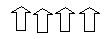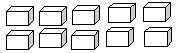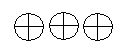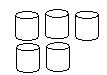Email us to get an instant 20% discount on highly effective K-12 Math & English kwizNET Programs!

#### Online Quiz (WorksheetABCD)

Questions Per Quiz = 2 4 6 8 10

### Grade 2 - Mathematics1.6 Even or Odd Using Pictures

 Method: Count the pictures given. Check if the total is odd or even. For a number to be odd, it has to end with 1, 3, 5, 7, or 9. For a number to be even, it has to end with 2, 4, 6, 8, or 0. Example:The number of patterns above is 8 . Answer : even Example:The number of patterns is 7 . Answer : odd Directions: Find if the number of pictures is even or odd. Draw 5 examples of your own and answer even or odd.
 Q 1: Count the number in the figure and answer even or odd.EvenOdd Q 2: Count the number in the figure and answer even or odd.EvenOdd Q 3: Count the number in the figure and answer even or odd.OddEven Q 4: Count the number in the figure and answer even or odd.EvenOdd Q 5: Count the number in the figure and answer even or odd.OddEven Q 6: Count the number in the figure and answer even or odd.OddEven Q 7: Count the number in the figure and answer even or odd.OddEven Q 8: Count the number in the figure and answer even or odd.EvenOdd Question 9: This question is available to subscribers only! Question 10: This question is available to subscribers only!